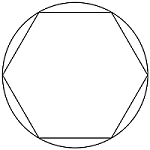# SAT Conceptual GeometryHow many lines of symmetry does the above figure have?

(A) $\ \$ Three
(B) $\ \$ Five
(C) $\ \$ Six
(D) $\ \$ Twelve
(E) $\ \$ Infinitely many

×